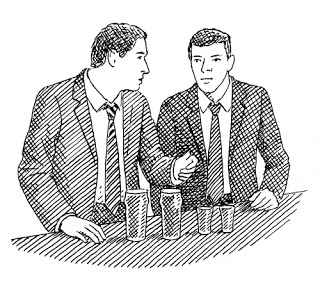# Winning Bar Bets With MathematicsBenford’s law is pretty neat. I first came across this phenomenon while watching a silly Internet TV show related to bar bets. The bar bet that exploits Benford’s law is a little complicated, and relies (of course) on the law of large numbers.

The premise is that you challenge your fellow bar patrons to a bet: that humans are no good at generating random numbers. It makes sense intuitively since people tend to pick numbers that have a special meaning, and there’s a finite number of numbers that have meaningful connotations (i.e. 3’s a charm, lucky number 7, etc.). To add to the challenge, you’ll throw in some randomness. Each person will come up with two randomly chosen quantities based on some data, such as the population of pet birds in the US and the volume of water in the Dead Sea in cubic feet. Then, multiply the two numbers to get a third number. The bet will be that even the first digit of *that* number is not uniformly distributed. On the surface, it seems counter-intuitive. But Benford’s law will give you an edge in this silly bar game.

It turns out that, given natural data, the first digit of any given number is not uniformly distributed across digits 1 through 9. Exponentially growing phenomena, for example, may double in size each period. Thus, the observed quantities of something like a growing bacteria colony would be 1, 2, 4, 8, 16, 32, 64, 128, 256, etc. Taking the first digit of these quantities, it’s clear that the lower digits have higher chances of occurring. The other way to prove it to yourself is to assume that for a random variable X with the first digit d, the digit d is equal to 1 if 1 <= d < 2, equal to 2 if 2 <= d < 3, etc. If we take the logarithm of each inequality, we end up with log 1 <= log d < log 2, log 2 <= log d < log 3, etc. In log-space, the size of each interval is actually non-uniform.

Thus, to win your bar game, assign one team of your bar patrons to the digits 1, 2, 3 and the other team to the digits 5, 6, 7, 8, 9. You, of course, want to make sure you’re on the team with digits 1, 2, 3. The team with the most “random” numbers that have the first digit belonging to their set wins. Assuming you play enough iterations, Benford’s law will ensure that you always win. By discarding the number 4, you give the illusion that you’re giving yourself even less of an advantage, when in fact it’s actually advantageous for you to simply disregard it rather than assign it to the other team.

In fact, an even more interesting fact about the tricky bar bet is that numbers that follow normal distributions don’t abide by Benford’s law. However, if you “mix” the numbers by way of multiplication, then the law reappears. So the seemingly innocuous trick of multiplying two arbitrary naturally occurring numbers just plays to your advantage.

Benford’s law can even be applied to fraud detection by analyzing the distribution of the first digit of numbers to look for anomalous results.